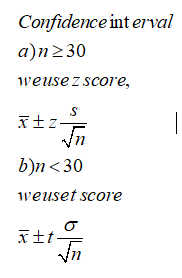# True or False? When calculating a confidence interval for one sample with a continuous outcome and the sample contains  fewer than 30 individuals, then a t-score should be used in place of a z-score.

Question
1 views

True or False? When calculating a confidence interval for one sample with a continuous outcome and the sample contains  fewer than 30 individuals, then a t-score should be used in place of a z-score.

check_circle

Step 1

Given that,

When calculating a confidence interval for one sample with a continuous outcome and the sample contains fewer than 30 individuals, then a t-score should be used in place of a z-score.

Step 2

Because, the formula for calculating confidence interval is depends on the size of the given sample.Step 3

Here they given that the sample contains fewer than...

### Want to see the full answer?

See Solution

#### Want to see this answer and more?

Solutions are written by subject experts who are available 24/7. Questions are typically answered within 1 hour.*

See Solution
*Response times may vary by subject and question.
Tagged in

### Statistics